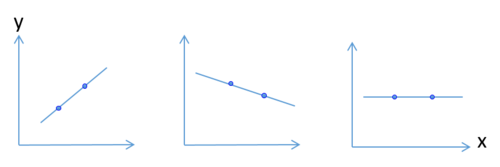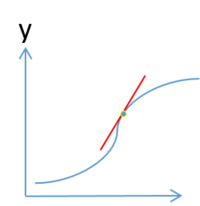# SMHS RateOfChange

(diff) ← Older revision | Latest revision (diff) | Newer revision → (diff)

## Scientific Methods for Health Sciences - Rate of Change

### Overview

Rate of change is a simple technical analysis indicator describing the rate in which one quantity changes in relation to another quantity:

$rate\, of\, change\,=\frac{change\, in\, y}{change\, in\, x\,}$

The rate of change can be positive or negative indicating an increase or decrease in y between two data points when x increases by a unit. When a quantity does not change over time, it implies a zero rate of change. Momentum is the absolute difference between two data points, which will correspond to the change in y. Here, we introduce the concept of rate of change and show application of rate of change in technical analysis.

### Motivation

Suppose we are studying on the changes in stock prices over a certain period and we know that the closing price on June 1, June 15, December 1, December 15 are 36, 38, 62, and 64 respectively. It is easily calculated that the absolute change in closing price of this stock from June 1 to June 15 is 38-36=2 which is the same as the absolute change in closing price of the stock from December 1 to December 15, which is also 64-62=2. However, is it enough to conclude that the price change is absolutely the same between these two time periods. For example, is it enough to reflect the same level of optimization for this stock in the market. The answer would be NO. Rate of change in the first half of June =(38-36)/36=0.0556, indicating that the stock price increased by 5.56% in the first half of June which is bigger compared to the rate of change in December (64-62)/62=0.0323. In order to make comparisons more comprehensively, we need to explore the properties of rate of change.

### Theory

#### Slope

To get a more visualized understanding of rate of change, we can think of slope as the simplest case of rate of change:

$rate\, of\, change\,=\frac{change\, in\, y}{change\, in\, x\,}$

which is the slope of the line located by the two data points. Zero rate of change will be illustrated with a horizontal line.#### Rate of change in a quadratic function

The rate of change at the point, which is located on the curve is indicated by the red line in the chart. The essence of this is that even the pattern of a point does not follow a linear pattern, the rate of change is can still be calculated at that specific time point where the change in x is almost zero, so the rate of change would be the instant change in y at that point.### Applications

• This article claimed that new applications which can utilize the process power available within the substation with substation computer systems dedicated to protection, control and data logging function in a substation. This paper cited the microcomputer based symmetrical component distance relay (SCDR), which facilitate real-time monitoring of positive sequence voltage phasor at the local power system bus. This paper describes the theoretical basis of regression analysis on frequency and rate-of-change of the frequency at the bus and presents the results of the experiments performed in AEP power system simulation laboratory. It also pointed out the plans for future field tests on the AEP system.
• This article conducted a linear regression analysis using the logarithm or the reciprocal of the serum creatinline concentration versus time to examine the rate of change of the serum creatinline concentration in 63 patients with chronic progressive renal disease of varied etiology. The relationship of 53 patients out of the total of 63 can be described by a single straight line and 5 patients had an accelerated rate of nephron destruction terminally (two slopes) regardless of the mathematical analysis. The remaining four patients had course changes either due to apparent spontaneous remissions or temporally related to therapy. The paper concluded that these data suggest that (functional) nephron loss in chronic progress disease is orderly and mathematically definable and the theoretical implications are that functional nephron loss is either exponential or constant.

### Software

In R: ratechange <- function (x) ((last(x)-first(x))/first(x))*100

### Problems

• Use the data in the table to find the rate of change of a patient vital sign. Then graph it.
x:_Time_(h) y: Vital_Sign_Measure
2 80
4 160
6 240